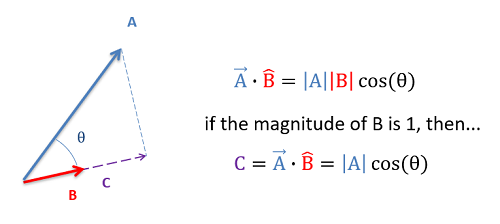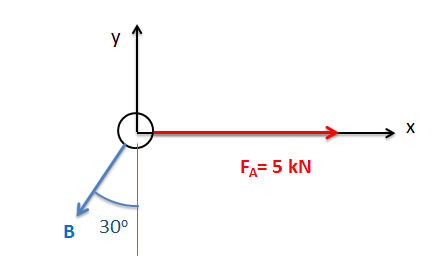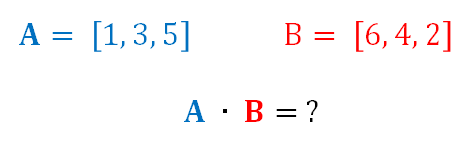﻿ Mechanics Map - Dot Product

# Dot Product

The dot product (also sometimes called the scalar product) is a mathematical operation that can be performed on any two vectors with the same number of elements. The result is a scalar number equal to the magnitude of the first vector, times the magnitude of the second vector, times the cosine of the angle between the two vectors.

 $\vec{A}\cdot \vec{B}= \left | A \right | \left | B \right | cos(\theta )$

In engineering mechanics, the dot product is used almost exclusively with a second vector being a unit vector. If the second vector in the dot product operation is a unit vector (thus having a magnitude of 1), the dot product will then represent the magnitude of the first vector in the direction of the unit vector. In this respect, a dot product is useful in determining the component of a given vector in any given direction, where the direction is given in terms of a unit vector.The dot product of a vector with a unit vector will give you the magnitude of the first vector in the direction of the unit vector.

As an alternative to the above equation for calculating the dot product, we can also calculate the dot product without knowing the angle between the vectors (theta). For this method we break each vector down into components and take the sum of each set of components multiplied together as shown in the equation below.

 $\vec{A}\cdot \vec{B}= \left( A_{x}*B_{x} \right )+\left( A_{y}*B_{y} \right )+\left( A_{z}*B_{z} \right )$

Finally, as with many vector operations, the true strength of the dot product is that computers can calculate them very quickly. Both MATLAB, and the Wolfram Vector Operation Calculator tool linked to in the left panel are able to computer dot products for you.

## Worked Problems:

### Question 1:

Find the dot product of force vector A with the unit vector shown with direction B.### Question 2:

Calculate the dot product of vector A with vector B by hand.### Question 3:

Calculate the dot product of vector A with vector B using MATLAB.### Question 4:

Calculate the dot product of vector A with vector B using the Wolfram Vector Operation Calculator.